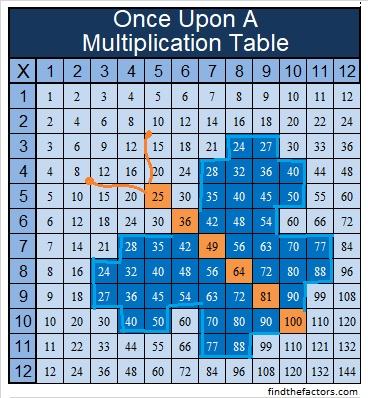# 73 and OnceIn Hungarian, “Multiplication Table” and “Times Table” are the same expression as “Once Upon a Time”. I very much enjoyed learning that when I went to the school of fairies today where even butterflies can learn to multiply. The author translates a sweet poem from Hungarian into English. I hope you will read the poem and remember its encouraging words whenever you try to solve one of my multiplication table puzzles or any other task that challenges you in life.

• 73 is a prime number.
• Prime factorization: 73 is prime.
• The exponent of prime number 73 is 1. Adding 1 to that exponent we get (1 + 1) = 2. Therefore 73 has exactly 2 factors.
• Factors of 73: 1, 73
• Factor pairs: 73 = 1 x 73
• 73 has no square factors that allow its square root to be simplified. √73 ≈ 8.5440037How do we know that 73 is a prime number? If 73 were not a prime number, then it would be divisible by at least one prime number less than or equal to √73 ≈ 8.5. Since 73 cannot be divided evenly by 2, 3, 5, or 7, we know that 73 is a prime number.

73 is never a clue in the FIND THE FACTORS puzzles.